#2019 iT 邦幫忙鐵人賽DAY 3
2
AI & Data

## Day03 從Pandas到Geopandas的幾種方法

Geopandas可以讀取各GIS格式進行空間運算，然而實際上我們可能拿到的資料不見得是GIS的格式

• 用Pandas讀取csv或Excel
• DataFrame轉為Geodataframe
• Geocoding

## 用Pandas讀取csv或Excel

``````import pandas as pd
``````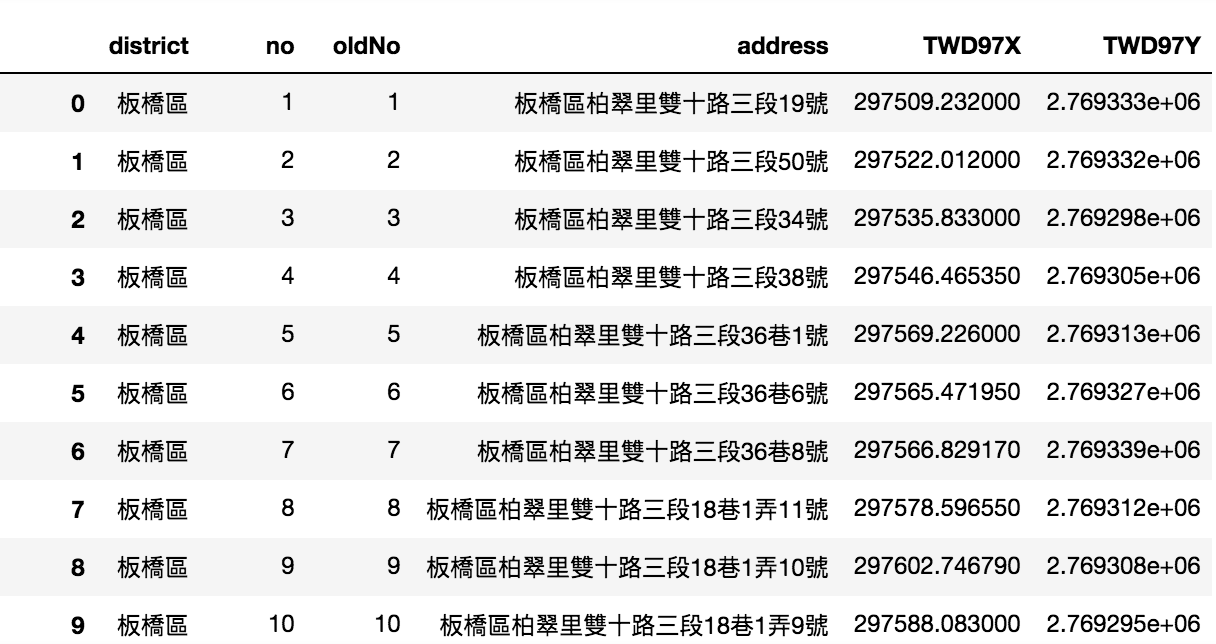ps.如果沒有pandas請先安裝

``````conda install pandas
``````

## DataFrame轉為Geodataframe

``````from shapely.geometry import Point
import geopandas as gpd
geom = [Point(xy) for xy in zip(df.TWD97X, df.TWD97Y)]
``````

[Day10] 坐標系統及WebGIS常用的坐標轉換

``````crs = {'init': 'epsg:3826'}
gdf = gpd.GeoDataFrame(df, crs=crs, geometry=geom)
gdf
``````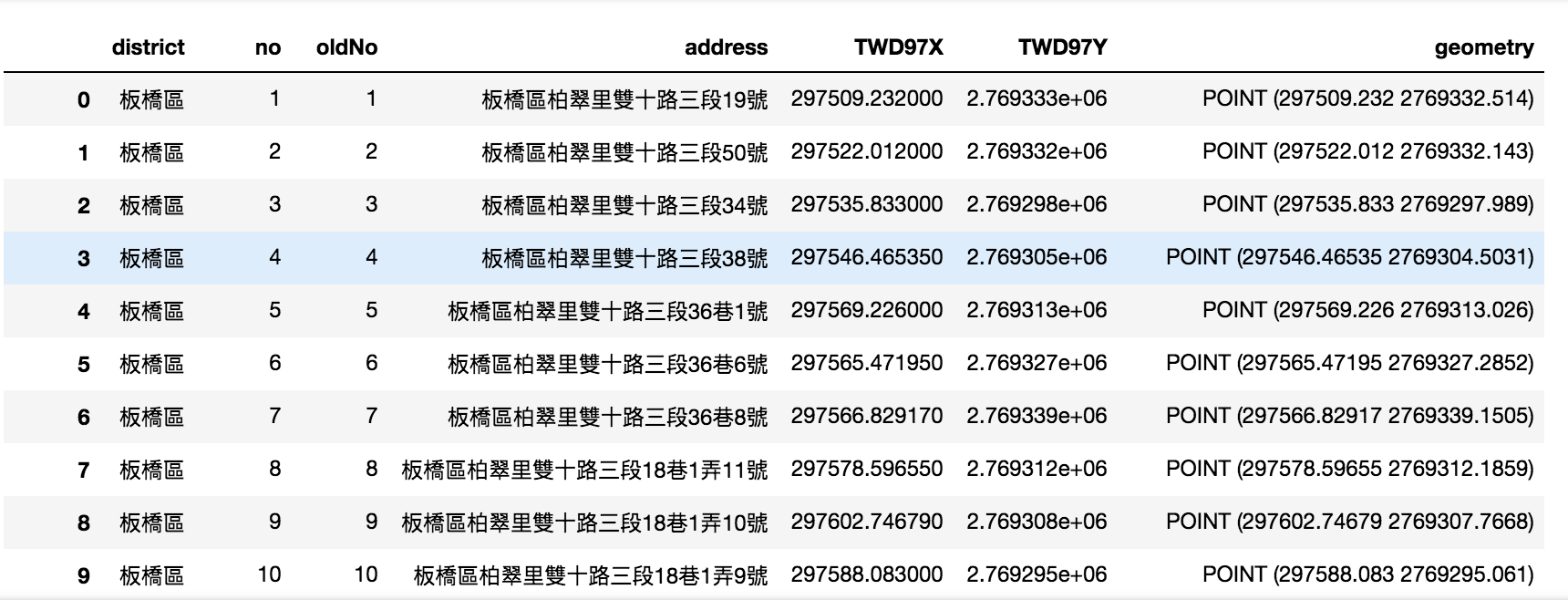``````gdf[gdf['district']=='永和區'].plot(column='address')
``````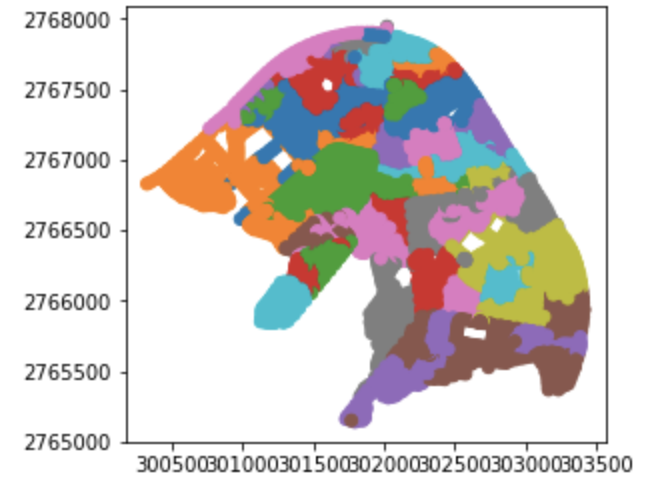## Geocoding

Geocoding會以TomTom Developer Portal Geocode提供的服務為例(個人經驗，這個服務的Geocoding會有較好的成果)

``````import pandas as pd
df
``````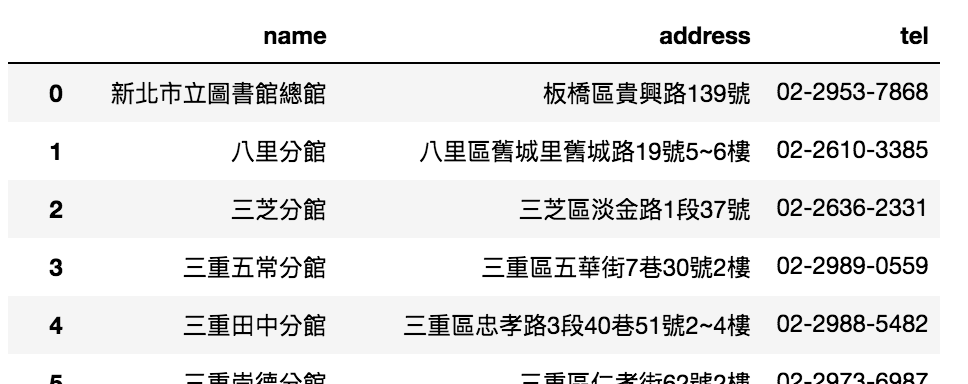api呼叫的方法及回應的格式直接參考TomTom Developer Portal Geocode的說明

``````import requests,json

url = 'https://api.tomtom.com/search/2/geocode/{}.json?&key=YOUR_KEY&countrySet=TWN&language=zh-TW&limit=1'.format(
response = requests.get(url)
data = response.text
return js['results']['position']
``````

ps. requests需要安裝，

``````conda install requests
``````

``````# 這邊使用df.head()測試避免一天request太多
``````

``````crs = {'init': 'epsg:4326'}
gdf
``````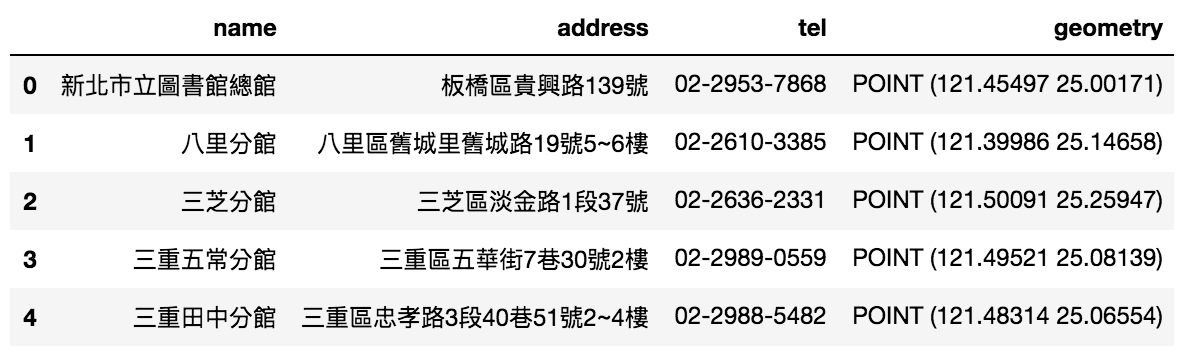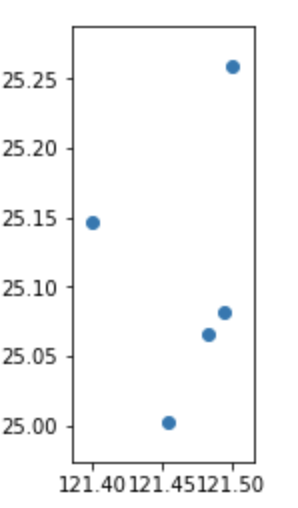GeoDataFrame也可以輸出成GIS格式，匯出在其他平台檢視與處理，包含常用的shp或是geojson

``````gdf.to_file(driver = 'ESRI Shapefile', filename = 'output/Light.shp')
``````